# Algorithmic worlds

## -

Back to the information page

Introduction
Algorithmic art
Pictorial algorithms
Ultra Fractal
Algorithmic worlds
Piling patterns
The structure
Pattern generators
Index operators
The piling operator
An example
Other modules

## Fixed edges pattern

This pattern is an early attempt to produce an "organic" pattern, in the same vein as Perlin noise.

Like most patterns, Fixed edges starts with a square gird. Recall that the pattern generators have to return a (real) number for each point of the plane. The idea here is to fix the value of the pattern on the edges of the gird by some random process, and interpolate inside the square tiles. This is a very general idea that I have not yet fully explored. In particular, it might be interesting to use tilings other than the regular square one. The current Fixed edges algorithm has two modes. In one of them, a periodic function is choosen for each edges, with a random amplitude and (integer) frequency. In the image below, the function is a sine.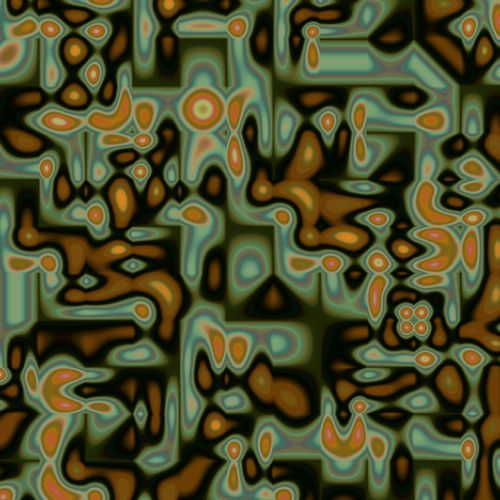There it is a squared sine: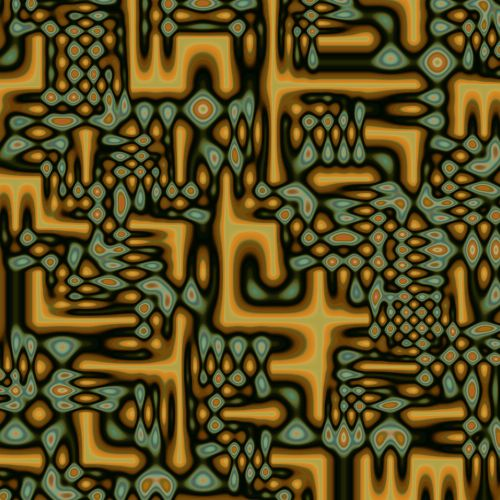and there a triangle wave: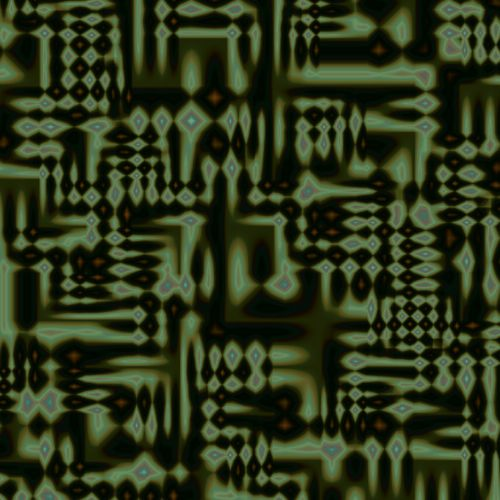The second mode chooses for each edge a sum of such periodic functions. Here is an example of the output, when the periodic function is a sine.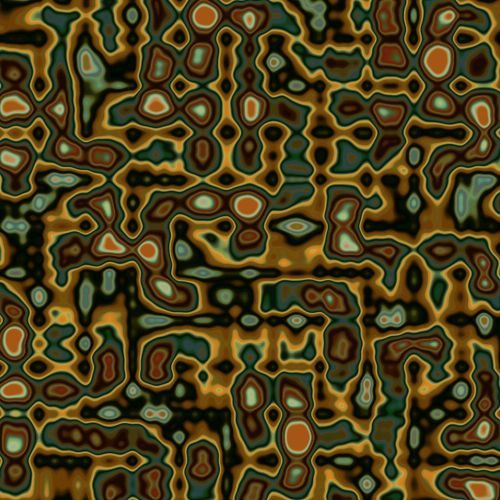An example after piling: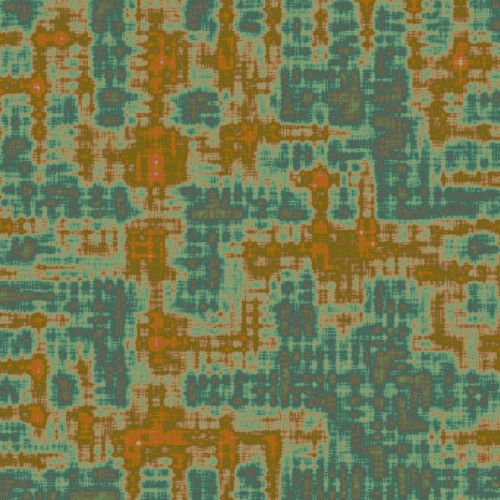Though it does produce smooth organic pattern, the underlying structure of the square gird remains noticeable. Works using this pattern can be found here.

 Copyright S.Monnier 2009-2019. Bookmark or share